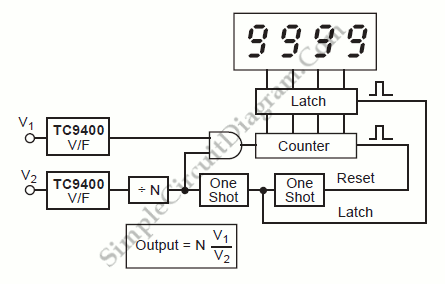# TC9400 Analog Division (Ratiometric Measurement)A circuit which will divide one analog signal by another is one of the most difficult circuits to build.  TC9400 voltage-to-frequency converter make it easy to build such circuit.  Here is the block diagram of the system:Two voltage-to-frequency (V/F) converters can do such division with ease. While the denominator forms the time base, the numerator is counted directly as a signal. [System’s block diagram source: Microchip Application Note]